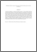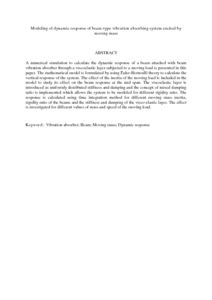# Modeling of dynamic response of beam-type vibration absorbing system excited by moving mass

## Citation

Putra, Azma and Y. Abd, Mothanna and Abdul Jalil, Nawal Aswan and Susilo, Sidik (2013) Modeling of dynamic response of beam-type vibration absorbing system excited by moving mass. International Review of Mechanical Engineering, 7 (5). pp. 813-820. ISSN 1970-8734

## Abstract

A numerical simulation to calculate the dynamic response of a beam attached with beam vibration absorber through a viscoelastic layer subjected to a moving load is presented in this paper. The mathematical model is formulated by using Euler-Bernoulli theory to calculate the vertical response of the system. The effect of the inertia of the moving load is included in the model to study its effect on the beam response at the mid span. The viscoelastic layer is introduced as uniformly distributed stiffness and damping and the concept of mixed damping ratio is implemented which allows the system to be modeled for different rigidity ratio. The response is calculated using time integration method for different moving mass inertia, rigidity ratio of the beams and the stiffness and damping of the visco-elastic layer. The effect is investigated for different values of mass and speed of the moving load.Preview
PDF (Abstract)
Modeling of dynamic response of beam.pdfView Item